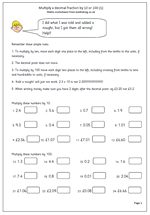# Maths worksheet: Multiply decimalsThere are some definite dos and don’ts when it comes to multiplying decimals ‘in your head’.

To multiply by 10 move each digit one place to the left, including tenths to the units, if necessary.

To multiply by 100 move each digit two places to the right.

The big don’t is to think that ‘add a nought’ is a short cut to multiplying by 10. 2.3 x 10 is not 2.30!!

This is a good check up page to make sure children understand what they are doing.

Multiply decimal fractions by 10 and 100 (1)